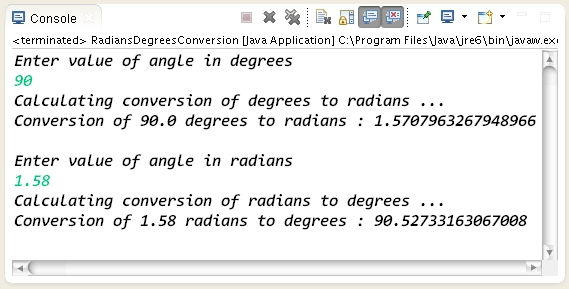How to convert degrees into radians and radians into degress using Math class in Java ?.

 Tweet Program to demonstrate how to convert degrees into radians and radians into degress using Math class in Java package com.hubberspot.code; import java.util.Scanner; public class RadiansDegreesConversion { public static void main(String[] args) { // Create a Scanner object which will read // values from the console which user enters Scanner scanner = new Scanner(System.in); // Getting input from user from the console System.out.println("Enter value of angle in degrees "); // Calling nextDouble method of scanner for // taking a double value from user and storing // it in degrees variable double degrees = scanner.nextDouble(); System.out.println("Calculating conversion of degrees to radians ... "); // In order to calculate conversion of degrees to radians // we use Math class toRadians() static method which takes // in a degree and returns back the converted value to radians double result = Math.toRadians(degrees); // printing the result on the console System.out.println("Conversion of " + degrees + " degrees to radians : " + result); System.out.println(); // Getting input from user from the console System.out.println("Enter value of angle in radians "); // Calling nextDouble method of scanner for // taking a double value from user and storing // it in radians variable double radians = scanner.nextDouble(); System.out.println("Calculating conversion of radians to degrees ... "); // In order to calculate conversion of radians to degrees // we use Math class toDegrees() static method which takes // in a radian and returns back the converted value to degrees result = Math.toDegrees(radians); // printing the result on the console System.out.println("Conversion of " + radians + " radians to degrees : " + result); } } Output of the program :Program to demonstrate how to convert degrees into radians and radians into degress using Math class in Java

package com.hubberspot.code;

import java.util.Scanner;

public class RadiansDegreesConversion {

public static void main(String[] args) {

// Create a Scanner object which will read
// values from the console which user enters
Scanner scanner = new Scanner(System.in);

// Getting input from user from the console
System.out.println("Enter value of angle in degrees ");

// Calling nextDouble method of scanner for
// taking a double value from user and storing
// it in degrees variable
double degrees = scanner.nextDouble();

System.out.println("Calculating conversion of degrees to radians ... ");

// In order to calculate conversion of degrees to radians
// we use Math class toRadians() static method which takes
// in a degree and returns back the converted value to radians
double result = Math.toRadians(degrees);

// printing the result on the console
System.out.println("Conversion of " + degrees + " degrees to radians : "
+ result);

System.out.println();

// Getting input from user from the console
System.out.println("Enter value of angle in radians ");

// Calling nextDouble method of scanner for
// taking a double value from user and storing
// it in radians variable
double radians = scanner.nextDouble();

System.out.println("Calculating conversion of radians to degrees ... ");

// In order to calculate conversion of radians to degrees
// we use Math class toDegrees() static method which takes
// in a radian and returns back the converted value to degrees

// printing the result on the console
System.out.println("Conversion of " + radians + " radians to degrees : "
+ result);

}

}

Output of the program :

Please Give Us Your 1 Minute In Sharing This Post!
SOCIALIZE IT →